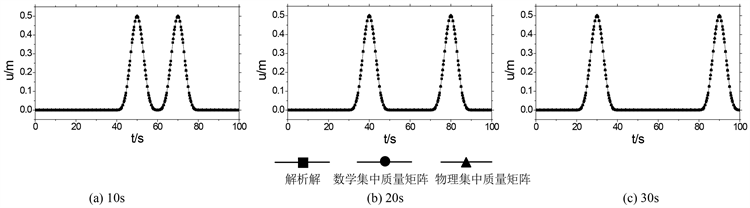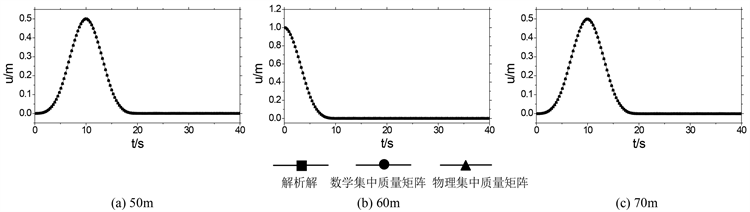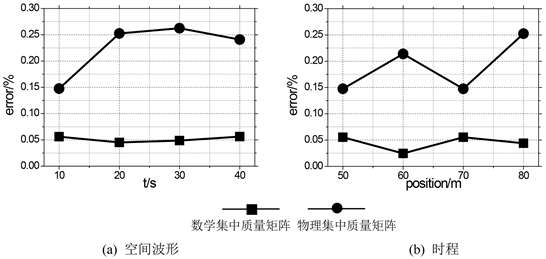﻿ Chebyshev谱元法模拟波动的两种集中质量矩阵

# Chebyshev谱元法模拟波动的两种集中质量矩阵Two Kinds of Lumped Mass Matrixes of Simulation of Wave Problem with Chebyshev Spectral Element Method

Abstract: Wave simulation is an important procedure for seismic analysis of engineering structure. Chebyshev Spectral Element Method is a kind of method to solve differential equations with high accuracy. Chebyshev Spectral Element Method has properties of high accuracy and high efficiency when it is used to simulate wave problem. The mass matrix of Chebyshev Spectral Element Method solving wave problem is consistent mass matrix which is space coupled. When it is used together with normal analysis procedure in time domain, it is time and space coupled. In each time step, it is needed to solve linear equations, and the efficiency is limited. In this paper, two kinds of lumped mass matrixes are derived based on Chebyshev Spectral Element Method consistent mass matrix. The math equations of the two mass matrixes are given. A procedure is given to solve one dimension wave problem. Chebyshev Spectral Element Method with the two matrixes is used in space domain, and central differential method is used in time domain. This procedure is decoupled in time and space domain. Numerical analysis shows that, this procedure has high accuracy, and it is not needed to solve linear equations in each time step, so compute efficiency can be largely enhanced. Within the two mass matrixes, math lumped mass matrix has higher accuracy than physical lumped mass matrix.

1. 前言

2. 波动模拟的Chebyshev谱元模型

$\rho \frac{{\partial }^{2}u}{\partial {t}^{2}}=\mu \frac{{\partial }^{2}u}{\partial {x}^{2}}$ (1)

$\underset{\Omega }{\int }\rho v\stackrel{¨}{u}\text{d}x+\underset{\Omega }{\int }\nabla v\mu \nabla u\text{d}x=0$ (2)

$\underset{\Omega }{\int }{\rho }^{e}v{\stackrel{¨}{u}}^{e}\text{d}x+\underset{\Omega }{\int }\nabla v{\mu }^{e}\nabla {u}^{e}\text{d}x=0$ (3)

${u}^{e}\left(x\right)=\underset{i=0}{\overset{N-1}{\sum }}{a}_{i}{A}_{i}\left(x\right)$ (4)

${\xi }_{i}=\mathrm{cos}\left(\frac{\pi i}{m}\right),\text{\hspace{0.17em}}i=0,1,\cdots ,m$ (5)

${u}^{e}\left(x\right)=\underset{i=0}{\overset{N-1}{\sum }}{u}^{e}\left({x}_{i}\right){l}_{i}\left(x\right)$ (6)

${M}^{e}{\stackrel{¨}{u}}^{e}\left(t\right)+{K}^{e}{u}^{e}\left(t\right)=0$ (7)

${M}_{ij}^{e}=\rho J\frac{4}{{N}^{2}{\stackrel{¯}{c}}_{i}{\stackrel{¯}{c}}_{j}}\underset{k,l=0}{\overset{N}{\sum }}\left(\frac{1}{{\stackrel{¯}{c}}_{k}{\stackrel{¯}{c}}_{l}}{T}_{k}\left({\epsilon }_{i}\right){T}_{l}\left({\epsilon }_{j}\right)\underset{-1}{\overset{1}{\int }}{T}_{k}\left(\epsilon \right){T}_{l}\left(\epsilon \right)\text{d}\epsilon \right)$ (8)

${K}_{ij}^{e}=J{\left({J}^{-1}\right)}^{2}\frac{4}{{N}^{2}{\stackrel{¯}{c}}_{i}{\stackrel{¯}{c}}_{j}}\underset{k,l=0}{\overset{N}{\sum }}\left(\frac{1}{{\stackrel{¯}{c}}_{k}{\stackrel{¯}{c}}_{l}}{T}_{k}\left({\epsilon }_{i}\right){T}_{l}\left({\epsilon }_{j}\right)\underset{-1}{\overset{1}{\int }}{{T}^{\prime }}_{k}\left(\epsilon \right){{T}^{\prime }}_{l}\left(\epsilon \right)\text{d}\epsilon \right)$ (9)

$J=\frac{\Delta x}{2}$${J}^{-1}=\frac{2}{\Delta x}$

$M\stackrel{¨}{u}\left(t\right)+Ku\left(t\right)=0$ (10)

3. 集中质量矩阵模型

3.1. 数学集中质量矩阵

$M=\left[\begin{array}{ccccc}0.0444& -0.0071& -0.0158& 0.0198& -0.0079\\ -0.0071& 0.2539& 0.0507& -0.0507& 0.0198\\ -0.0158& 0.0507& 0.3301& 0.0507& -0.0158\\ 0.0198& -0.0507& 0.0507& 0.2539& -0.0071\\ -0.0079& 0.0198& -0.0158& -0.0071& 0.0444\end{array}\right]$

${M}_{s}\left(i,i\right)=\underset{j=1}{\overset{N}{\sum }}M\left(i,j\right),\text{\hspace{0.17em}}\text{\hspace{0.17em}}i=1,2,\cdots ,N;j=1,2,\cdots ,N$ (11.a)

${M}_{s}\left(i,j\right)=0,\text{\hspace{0.17em}}\text{\hspace{0.17em}}i=1,2,\cdots ,N;j=1,2,\cdots ,N;i\ne j$ (11.b)

3.2. 物理集中质量矩阵

${M}_{w}\left(i,i\right)=\frac{{x}_{i+1}-{x}_{i-1}}{2}\rho ,i=2,\cdots ,N-1$ (12.a)

${M}_{w}\left(i,i\right)=\frac{{x}_{2}-{x}_{1}}{2}\rho ,i=1$ (12.b)

${M}_{w}\left(i,i\right)=\frac{{x}_{N}-{x}_{N-1}}{2}\rho ,i=N$ (12.c)

${M}_{w}\left(i,j\right)=0,i=1,2,\cdots ,N;j=1,2,\cdots ,N;i\ne j$ (12.d)

${M}_{s}\stackrel{¨}{u}\left(t\right)+Ku\left(t\right)=0$ (13)

${M}_{w}\stackrel{¨}{u}\left(t\right)+Ku\left(t\right)=0$ (14)

4. 时空解耦模拟方案

${M}_{s}{\stackrel{¨}{u}}_{i}\left(t\right)+K{u}_{i}\left(t\right)=0$ (15)

${\stackrel{¨}{u}}_{i}=\frac{{u}_{i+1}-2{u}_{i}+{u}_{i-1}}{\Delta {t}^{2}}$ (16)

${M}_{s}\frac{{u}_{i+1}-2{u}_{i}+{u}_{i-1}}{\Delta {t}^{2}}+K{u}_{i}=0$ (17)

$\frac{1}{\Delta {t}^{2}}{M}_{s}{u}_{i+1}=-\left(K{u}_{i}-\frac{2}{\Delta {t}^{2}}{M}_{s}\right){u}_{i}-\frac{2}{\Delta {t}^{2}}{M}_{s}{u}_{i-1}$ (18)

5. 数值实验

$P\left(\tau \right)=16\left[G\left(\tau \right)-4G\left(\tau -\frac{1}{4}\right)+6G\left(\tau -\frac{1}{2}\right)-4G\left(\tau -\frac{3}{4}\right)+G\left(\tau -1\right)\right]$ (19)

$G\left(\tau \right)={\tau }^{3}H\left(\tau \right)$ (20)Figure 1. Spatial waveform comparisonFigure 2. Comparison of displacement time historyFigure 3. Comparison of peak relative error

6. 结论

1) 两种集中质量矩阵都可以很好地模拟波动的传播过程，且具有较高的计算精度。

2) 数学集中质量矩阵的计算精度高于物理集中质量矩阵。在本文给定参数下，数学集中质量矩阵的相对误差约为物理集中质量矩阵的1/5~1/3。

3) 采用本文提出的两种集中质量矩阵的谱元法结合时间域中心差分法可以使得计算过程成为一个时空解耦的过程，不需每时步联立求解线性方程组，可以大幅度提高计算效率。

 Shen, J. and Tang, T. (2007) Spectral and High-Order Methods with Applications. Science Press (Beijing), Beijing.

 Patera, A.T. (1984) A Spectral Element Method for Fluid Dynamics: Laminar Flow in a Channel Expansion. Computational Physics, 54, 468-488.
https://doi.org/10.1016/0021-9991(84)90128-1

 Funaro, D. (1988) Domain Decomposition Methods for Pseudospectral Approximations. Part I, Second Order Equations in One Dimension. Numerische Mathematik, 52, 329-344.
https://doi.org/10.1007/BF01398883

 Bernadi, C., Girault, V. and Maday, Y. (1992) Mixed Spectral Element Approximation of the Navier-Stokes Equations in the Stream-Function and Vorticity Formulation. IMA Journal of Numerical Analysis, 12, 565-608.
https://doi.org/10.1093/imanum/12.4.565

 Seriani, G. and Priolo, E. (1994) Spectral Element Method for Acoustic Wave Simulation in Heterogeneous Media. Finite Element in Analysis and Design, 16, 337-348.
https://doi.org/10.1016/0168-874X(94)90076-0

 王秀明, Seriani, G., 林伟军. 利用谱元法计算弹性波场的若干理论问题[J]. 中国科学G辑, 2007, 37(1): 41-59.

 Seriani, G. (1998) 3-D Large-Scale Wave Propagation Modeling by Spectral Element Method on Cray T3E Multiprocessor. Journal of Computer Methods in Applied Mechanics and Engineering, 164, 235-247.
https://doi.org/10.1016/S0045-7825(98)00057-7

 Igawa, H., Komatsu, K., Yamaguchi, I. and Kasai, T. (2004) Wave Propagation Analysis of Frame Structures Using the Spectral Element Method. Journal of Sound and Vibration, 277, 1071-1081.
https://doi.org/10.1016/j.jsv.2003.11.026

 Rong, Z. and Xu, C. (2008) Numerical Approximation of Acoustic Waves by Spectral Element Methods. Journal of Applied Numerical Mathematics, 58, 999-1016.
https://doi.org/10.1016/j.apnum.2007.04.008

 Kim, A.D. and Ishimaruy A. (1999) A Chebyshev Spectral Method for Radiative Transfer Equations Applied to Electromagnetic Wave Propagation and Scattering in a Discrete Random Medium. Journal of Computational Physics, 152, 264-280.
https://doi.org/10.1006/jcph.1999.6247

 廖振鹏. 工程波动理论导论[M]. 北京: 科学出版社, 2002.

Top